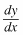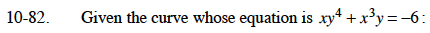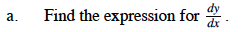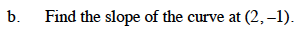### Home > CALC > Chapter 10 > Lesson 10.1.8 > Problem10-82

10-82.
1. Given the curve whose equation is xy4 + x3 y = −6: Homework Help ✎

1. Find the expression for.

2. Find the slope of the curve at (2, −1).Evaluate your equation in part (a) for x = 2, y = –1.